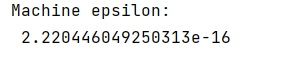# NumPy Machine Epsilon

Learn, how to get the numpy machine epsilon format of data?
Submitted by Pranit Sharma, on December 27, 2022

NumPy is an abbreviated form of Numerical Python. It is used for different types of scientific operations in python. Numpy is a vast library in python which is used for almost every kind of scientific or mathematical operation. It is itself an array which is a collection of various methods and functions for processing the arrays.

## Machine Epsilon in Python NumPy

Machine epsilon is suitable for double-precision numbers. We need to modify it in such a way that it also supports single precision.
An easier way to get the machine epsilon for a given float type is to use a special method provided by NumPy called numpy.finfo().

Let us understand with the help of an example,

## Python code to demonstrate the example of NumPy Machine Epsilon

```# Import numpy
import numpy as np

# Print the machine epsilon
print("Machine epsilon:\n",np.finfo(float).eps)
```

Output: